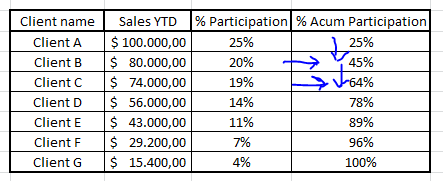Qlik Sense App Development

Discussion board where members can learn more about Qlik Sense App Development and Usage.

Announcements
Highlighted
New Contributor II

Problems to see percentage of participation per client and acumulate it.

Hi everyone ,

I have problems to calculate  "% of participation per client"

The calculation what i use is:

SUM({<[Sales YTD]={1}>}[Net Sales])/SUM({<[Sales YTD]={1},[Client Name]=>}[Net Sales])

If i divide the metric in two:

• SUM({<[Sales YTD]={1}>}[Net Sales])

Show net sale year to date based in selection

• SUM({<[Sales YTD]={1},[Client Name]=>}[Net Sales])

Show total net sale year to date ignoring selections ( In this case total net sales)

But when i put this two metrics in a table with Clients, both metrics show the same number and of course if i use the firsth complete metric the result is always 1.

My objective with that is obtain the "% of participation per client" acumulated.Is this posible ?

Tags (3)
6 Replies
Contributor

Re: Problems to see percentage of participation per client and acumulate it.

Hi Alvaro Garcia,

Please have a look at the attached qvw file and let me know if it works for you.

Thanks

Mouna ChandraPartner

Re: Problems to see percentage of participation per client and acumulate it.

There's nothing inside that .qvw except an inline table?

Contributor

Re: Problems to see percentage of participation per client and acumulate it.

I am sorry, I am not sure why you are not seeing the table in front end.

I am using the below measure to calculate the % accumulation.

=rangesum(above(sum([Sales YTD]),0,RowNo()))/sum(total [Sales YTD]).

Please this use this as a measure in you expressions it will give you the percentage.

Thanks

Mouna

Valued Contributor

Re: Problems to see percentage of participation per client and acumulate it.

Hi

I think using ABOVE function to take the previous percentage and add the percentage of the current row.

Maybe this:

• above([Acummulate]) + SUM({<[Sales YTD]={1}>}[Net Sales])/SUM({<[Sales YTD]={1},[Client Name]=>}[Net Sales])
Valued Contributor

Re: Problems to see percentage of participation per client and acumulate it.

Better if you use RangeSum as mounachandra1 said

RangeSum(Above(Sum([Sales YTD]),0,RowNo()))/Sum(total [Sales YTD])

Here is the example:Accumulations

Contributor

Re: Problems to see percentage of participation per client and acumulate it.

Can you please close the threat by marking the correct answer, so that might be helpful for others.

Thanks

Mouna Chandra